# 还记得2048怎么玩吗？快来玩会儿(摸鱼)吧！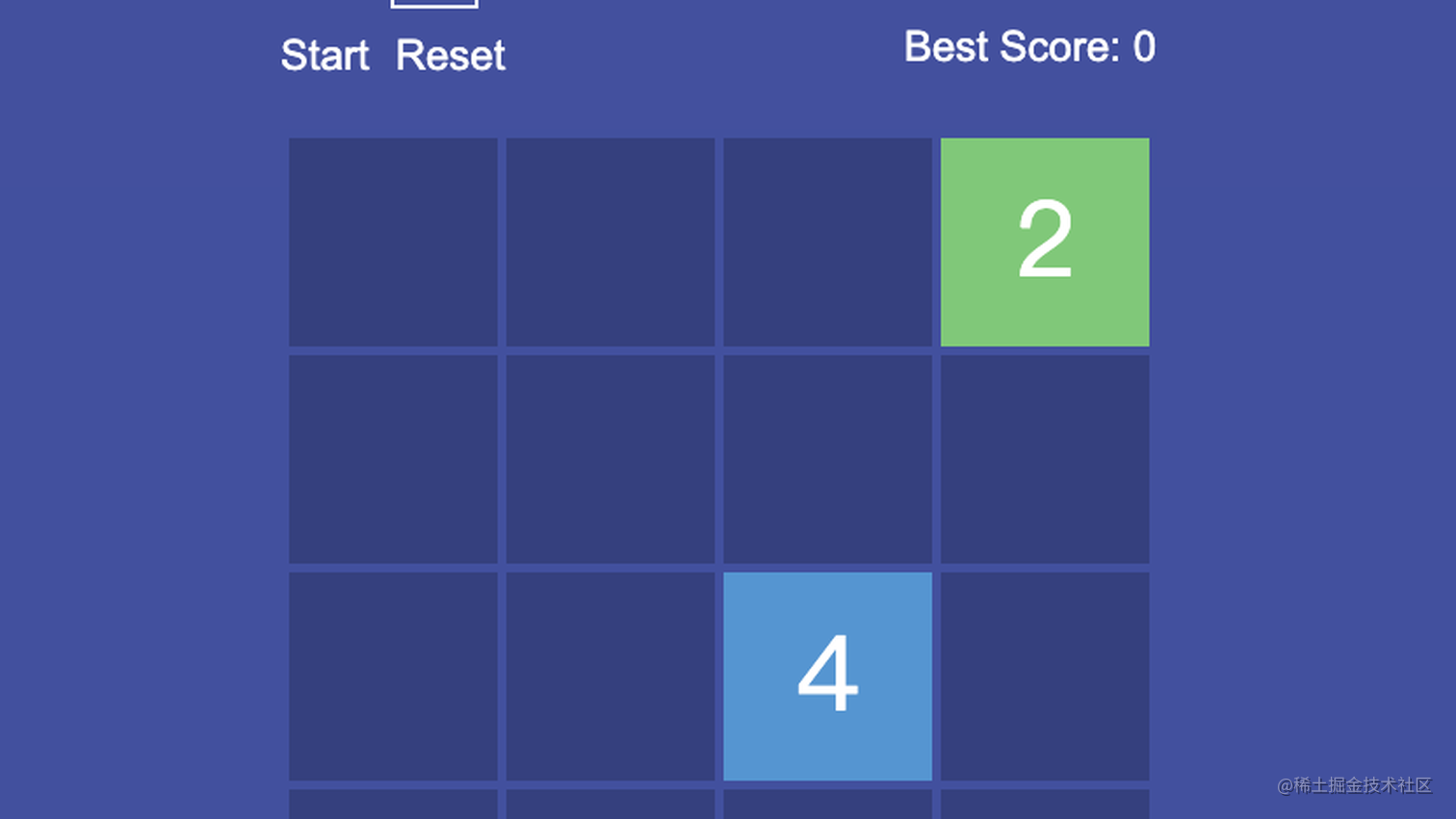### 2048 试玩
### 基础架子

``````<div class='container'>
<div class='fluid'>
<h1>2048</h1>
<div class='edits'>
<div class='size-block'>
<div class='set-size'>
<p id='size-title'>Size: </p>
<input id='size' type='number' value='4'>
</div>
<div class='btns'>
<div class='btn start'>Start</div>
<div class='btn reset'>Reset</div>
</div>
</div>
<div class='scores'>
<span id='score'>Score: 0</span>
<span id='bestScore'>Best Score: 0</span>
</div>
</div>
<div id='canvas-block'>
<div class='start2'>&gt; Start &lt;</div>
<div class='lose'>Game Over!</div>
<canvas id='canvas' width='500' height='500'></canvas>
</div>
</div>
</div>

``````...
#canvas{
background: rgba(var(--blue),1);
margin-top: 30px;
box-sizing: border-box;
}
h1{
font-size: 58px;
color: white;
margin-top: 30px;
}
.scores{
width: 50%;
display: flex;
flex-direction: column;
justify-content: center;
align-items: flex-end;
}
.edits{
min-width: 500px;
width: 100%;
display: flex;
flex-direction: row;
justify-content: space-between;
align-content: center;
margin-top: 30px;
font-size: 24px;
}
.set-size{
width: 100%;
display: flex;
justify-content: flex-start;
align-items: center;
flex-direction: row;
}
.size-block{
display: flex;
flex-direction: column;
width: 50%;
justify-content: center;
align-items: flex-start;
}
.btns, #bestScore{
margin-top: 15px;
}
.size-block input{
margin-left: 10px;
max-width: 50px;
}
#size{
background: transparent;
border: 2px solid white;
color: white;
font-size: 18px;
width: 60px;
outline: none;
box-sizing: border-box;
text-align: center;
}
.lose, .start2{
z-index: 999;
position: absolute;
margin-top: 15px;
font-size: 35px;
display: none;
}
.start2{
display: block;
min-width: 150px;
margin-top: 200px;
cursor: pointer;
}
#canvas-block{
position: relative;
display: flex;
justify-content: center;
align-items: center;
}
.btns{
display: flex;
flex-direction: row;
justify-content: flex-start;
align-items: center;
cursor: pointer;
}
.reset{
margin-left: 15px;
}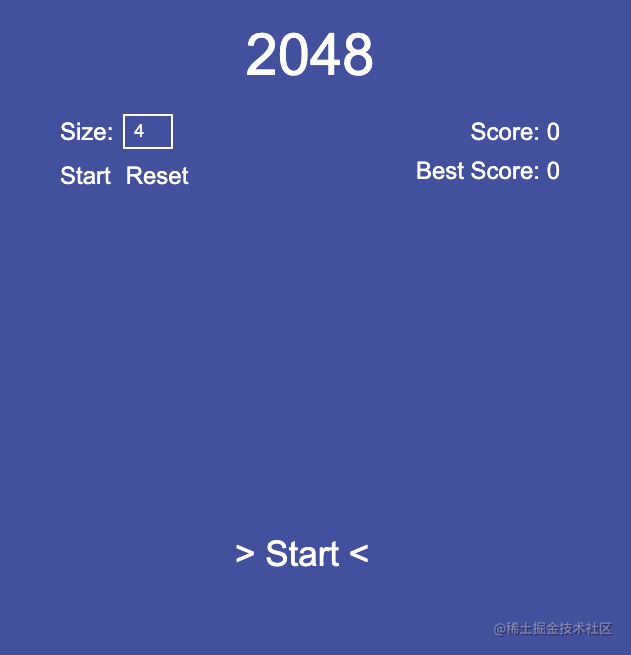### js + canvas 实现

``````class Game {
constructor() {
this.canvas = document.getElementById('canvas');
this.ctx = this.canvas.getContext('2d');
this.sizeInput = document.getElementById('size');
this.startBtn = document.querySelector('.start');
this.startBtn2 = document.querySelector('.start2');
this.scoreLabel = document.getElementById('score');
this.resetBtn = document.querySelector('.reset');
this.lessHtml = document.querySelector('.lose');
this.scoreValue = 0;
this.bestScore = document.getElementById('bestScore');
this.bestScoreValue = localStorage.getItem('score2048');
this.size = 4;
this.width = this.canvas.width / this.size - 6;
this.cells = [];
this.fontSize = 0;
this.loss = false;
}
}

``````...

init() {
this.publicEvent();
});

this.scoreValue = 0;
this.canvas.style.opacity = '1';
this.loss = false;
this.lessHtml.style.display = 'none';
this.bestScoreValue = localStorage.getItem('score2048');
this.scoreLabel.innerHTML = `Score: \${+this.scoreValue}`;
this.startGame();
this.initScore();
});
}

initState() {
this.initStart();

this.initScore();

this.initEvent();
}

initStart() {
this.canvas.style.display = 'none';
this.publicEvent();
});
}

publicEvent() {
if (this.sizeInput.value >= 3 && this.sizeInput.value <= 10) {
this.size = this.sizeInput.value;
this.width = this.canvas.width / this.size - 6;
this.canvasClear();
this.startGame();
this.canvas.style.display = 'block';
this.startBtn2.style.display = 'none';
} else {
return;
}
}

...

``````class Cell {
constructor(row, col, width) {
this.value = 0;
this.x = col * width + 5 * (col + 1);
this.y = row * width + 5 * (row + 1);
}
}

``````async createCells() {
for (let i = 0; i < this.size; i++) {
this.cells[i] = [];
for (let j = 0; j < this.size; j++) {
this.cells[i][j] = new Cell(i, j, this.width);
}
}
}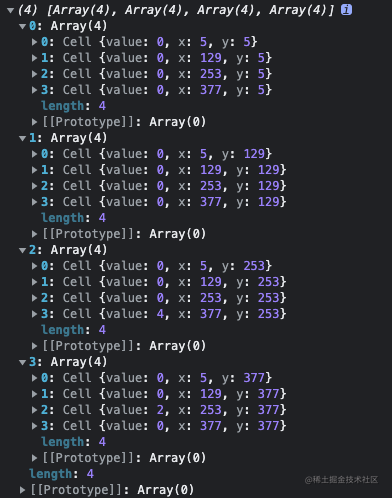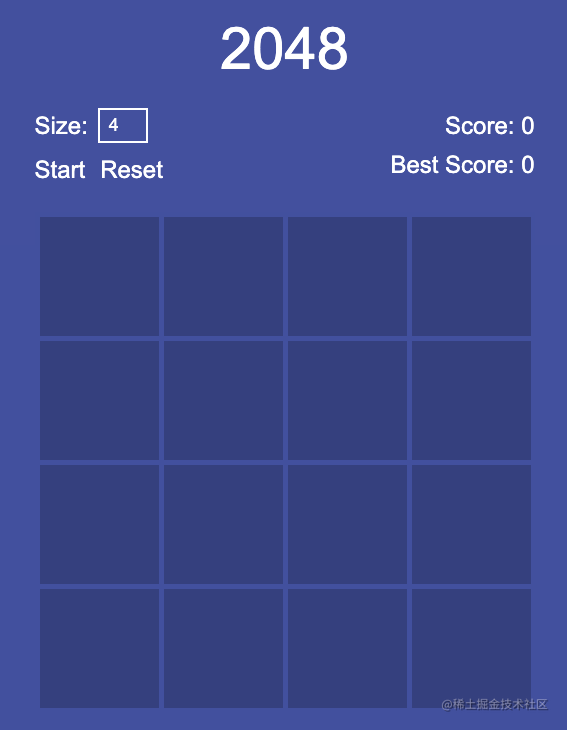``````...other code

async drawAllCells() {
for (let i = 0; i < this.size; i++) {
for (let j = 0; j < this.size; j++) {
this.drawCell(this.cells[i][j]);
}
}
}

...other code

``````drawCell(cell) {
this.ctx.beginPath();
this.ctx.rect(cell.x, cell.y, this.width, this.width);
this.ctx.fillStyle = "#384081";
this.ctx.fill();
}

`````` async startGame() {
await this.createCells();
await this.drawAllCells();
await this.pasteNewCell();
await this.pasteNewCell();
}

``````async pasteNewCell() {
let countFree = 0;
for (let i = 0; i < this.size; i++) {
for (let j = 0; j < this.size; j++) {
if (!this.cells[i][j].value) {
countFree++;
}
}
}

if (!countFree) {
this.finishGame();
return;
}

while (true) {
let row = Math.floor(Math.random() * this.size);
let col = Math.floor(Math.random() * this.size);

if (!this.cells[row][col].value) {
this.cells[row][col].value = 2 * Math.ceil(Math.random() * 2);
this.drawAllCells();
return;
}
}
}

pasteNewCell 内部，我们通过不断的循环来生成新的游戏方法，其中最主要的就是判断当前小方块的值是否为 0，如果不为 0，就会画一个新的方块展示在游戏区域内，反之则不执行。

``````drawCell(cell) {
this.ctx.beginPath();
this.ctx.rect(cell.x, cell.y, this.width, this.width);
this.ctx.fillStyle = "#384081";
this.ctx.fill();

if (cell.value) {
this.ctx.fillStyle = `\${this.cellColor(cell.value)}`;
this.ctx.fill();
this.fontSize = this.width / 2;
this.ctx.font = this.fontSize + 'px Viga';
this.ctx.fillStyle = 'white';
this.ctx.textAlign = "center";
this.ctx.fillText(cell.value, cell.x + this.width / 2, cell.y + this.width / 1.5);
}
}

cellColor(value) {
const colorList = new Map([
[0, 'rgb(135,200,116)'],
[2, 'rgb(135,200,116)'],
[4, 'rgb(95,149,212)'],
[8, 'rgb(139,89,177)'],
[16, 'rgb(229,195,81)'],
[32, 'rgb(202,77,64)'],
[64, 'rgb(108,129,112)'],
[128, 'rgb(207,126,63)'],
[256, 'rgb(82,125,124)'],
[512, 'rgb(191,76,134)'],
[1024, 'rgb(119,41,92)'],
[2048, 'rgb(118,179,194)'],
[4096, 'rgb(52,63,79)'],
]);

return colorList.get(value) || 'rgba(70,80,161,0.8)';
}

``````initEvent() {
if (!this.loss && this.canvas.style.display === 'block') {
switch (event.key) {
case 'ArrowUp':
this.moveUp();
break;
case 'ArrowDown':
this.moveDown();
break;
case 'ArrowLeft':
this.moveLeft();
break;
case 'ArrowRight':
this.moveRight();
break;
}
this.scoreLabel.innerHTML = `Score: \${+this.scoreValue}`;
}
});
}

``````...other code

moveUp() {
let row;
for (let j = 0; j < this.size; j++) {
for (let i = 1; i < this.size; i++) {
if (this.cells[i][j].value) {
row = i;
while (row > 1) {
if (!this.cells[row - 1][j].value) {
this.cells[row - 1][j].value = this.cells[row][j].value;
this.cells[row][j].value = 0;
row--;
} else if (this.cells[row][j].value === this.cells[row - 1][j].value) {
this.cells[row - 1][j].value *= 2;
this.scoreValue += this.cells[row - 1][j].value;
this.cells[row][j].value = 0;
break;
} else {
break;
}
}
}
}
}
this.pasteNewCell();
}

moveDown() {
let row;
for (let j = 0; j < this.size; j++) {
for (let i = this.size - 2; i >= 0; i--) {
if (this.cells[i][j].value) {
row = i;
while (row + 1 < this.size) {
if (!this.cells[row + 1][j].value) {
this.cells[row + 1][j].value = this.cells[row][j].value;
this.cells[row][j].value = 0;
row++;
} else if (this.cells[row][j].value === this.cells[row + 1][j].value) {
this.cells[row + 1][j].value *= 2;
this.scoreValue += this.cells[row + 1][j].value;
this.cells[row][j].value = 0;
break;
} else {
break;
}
}
}
}
}
this.pasteNewCell();
}

...other code

``````finishGame() {
const currentScore = localStorage.getItem('score2048');

if (currentScore < this.scoreValue) {
localStorage.setItem('score2048', this.scoreValue);
}
this.canvas.style.opacity = '0.3';
this.loss = true;
this.lessHtml.style.display = 'block';
}